# Stability of Horizontal Porous Layer Heated from Below Using FORCHHEIMER’S Model

Stability of Horizontal Porous Layer Heated from Below Using FORCHHEIMER’S Model

A. Al-Ameen H. M. Duwairi

Mechanical Engineering Department, Faculty of Engineering and Technology, The University of Jordan, 11942 Amman, Jordan

Corresponding Author Email:
duwairi@ju.edu.jo
Page:
109-114
|
DOI:
http://doi.org/10.18280/ijht.330316
N/A
|
Accepted:
N/A
|
Published:
30 September 2015
| Citation

OPEN ACCESS

Abstract:

In this present study the stability of horizontal porous layer heated from below using Forchheimer’s model is studied numerically. A uniform heat is applied for heating and cooling the two opposing walls of the enclosure while the other two walls are adiabatic. The Forchheimer extension of Darcy and energy equations are going to be transformed into dimensionless form using a set of suitable variables then solved using a finite difference scheme. Governing parameters are the Forchheimer number and the Rayleigh number. The results show that both the Forchheimer’s number and the Rayleigh number have significant effect on the stability analysis in porous medium.

Keywords:

Stability, Natural convection, Porous media.

1. Introduction

The research of natural convection in porous medium has been conducted widely in recent years, which involves post-accidental heat removal in nuclear reactors, cooling of radioactive waste containers, the migration of moisture through the air contained in fibrous insulations, the dispersion of chemical pollutants through water-saturated materials, heat exchangers, solar power collectors, grain storage, food processing, energy efficient drying process.

There are many open literature related to natural convection in horizontal porous layer. The heat convection of porous media was studied from a very long time by Horton and Rogers , Duwairi , Duwairi and Al-Kablawi , Duwairi et al. , the main target and purpose was to understand fluid motion of convection stream line in deep porous layers. A linear stability analysis for the problem was the main concern of Horton and Roger, they found the value of  critical Rayleigh number for porous medium equal to Ra=40 which indicate the transform from conduction to convection heat transfer state of the fluid in porous media. It means that the convection heat transfer start to occur when Rayleigh number reaches that critical value. The stability problem for porous media also has been studied by Lapwood , who also found the same value for critical Rayleigh number and the same result for convection heat transfer. From that time a large amount of studies examining the various terms that affect the results of the convection heat transfer in porous media. Katto and Masuoka  found that the critical Rayleigh number is equal to 42. This result was obtained for a wide range for a porous media, the saturation fluid in their experiments was compressed nitrogen in order to decrease the temperature difference between the upper and lower surfaces required for creating large Rayleigh numbers .The main target of the experiments was to find whether parameters such as the size of the porous material, the porosity of the layer, and the solid-to-fluid conductivity ratio affect the critical Rayleigh number. Chen and Chen  Use the modified form of Darcy’s equation which included the Brinkman and Forchheimer terms in order to study the effect for viscous and inertial effects. Also they create a computational analysis in order to study the heat transfer.

Computational analysis in order to study the heat transfer Heat and Technology Prasad . Proposed new experiments to study the effect of Prandtl number and different solid-fluid conductivity ratios, Acrylic, aluminum, and glass spheres are used for the porous bed, silicone oils and ethylene glycol are used as the saturating fluids in the experiments. It was found that the relationship between porous layer height and Nusselt number remains constant with a variation in thermal conductivity ratio and/or the Prandtl number. Also, he found that by increasing the thermal conductivity of the solid matrix the heat transfer also is increased. Further, it was found that the composite fluid and porous layer have more ability to transport fluid compared to the pure fluid layer. Steven  studied the heat transfer characteristics of a fluid superposed porous layer system heated from below experimentally. Four different values of heat ratio to measure heat transfer rates are used. A cylindrical test chamber is used with 6 mm diameter glass beads as the porous layer and water as the saturating fluid. Temperature fluctuations within the system at various radial and vertical locations are observed. It was also found that for a given Rayleigh number, the overall Nusselt number for a pure fluid layer is lower than that for a composite fluid and porous layer, which indicated that the overlying layer enhanced the overall rate of heat transfer. However, the overall Nusselt number does not change significantly with heat ratio for different Rayleigh numbers

The main target of this study is to find the stability solution for the horizontal porous layer heated uniformly from below using different models start with Darcy model and Forchheimer’s model. Stability which means to find and determine the state of heat transfer when the heat transfer of fluid and plate will be convection or conduction in porous media also determine under what condition fluid motion first occurs. In this study a formulation of the stability problem is going to be done. The solution of stability analysis of this problem is a numerical solution since its mathematical formulation is a non-linear partial differential problem .An important variation of this problem is that of natural convection in a horizontal system that consists of a porous layer that is heated from below using Forchheimer’s model geophysical perspective.

2. Prolem Formulation

The considered geometry is an inclined rectangular enclosure, which is embedded in a fluid-saturated porous medium as shown in Fig. 1. One wall of the enclosure is kept at heat flux q1 and the opposite is kept at a heat flux q2. The other two walls of the enclosure are adiabatic, i.e., it is assumed that no heat is transferred into or out of walls.

## 1.png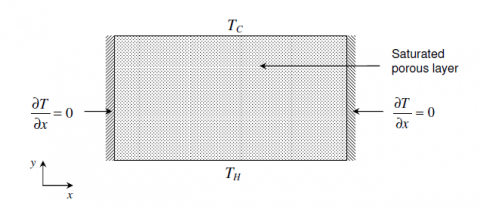Figure 1. Schematic of the problem of natural convection in a horizontal porous layer heated uniformly from below.

$\frac{\partial_{u}}{\partial_{x}}+\frac{\partial_{v}}{\partial_{y}}=0$  (1)

The momentum equation in x and y-directions:

$\frac{\mu \phi}{K}_{u+} \frac{\rho \phi F}{\sqrt{K}} u^{2}=-\frac{\partial p}{\partial x}$    (2)

$\frac{\mu \phi}{K} \mathrm{V}+\frac{\rho \phi \mathrm{F}}{\sqrt{K}} v^{2}=-\frac{\partial p}{\partial y}+\beta g p_{f}\left(T-T_{c}\right)$   (3)

The energy equation:

$\sigma \frac{\partial T}{\partial t}+\mathrm{u} \frac{\partial T}{\partial x}+\mathrm{v} \frac{\partial T}{\partial y}=\alpha_{a}\left(\frac{\partial^{2} T}{\partial x^{2}}+\frac{\partial^{2} T}{\partial y^{2}}\right)$    (4)

Where x and y are the horizontal and vertical coordinates, and the corresponding velocities are u and v respectively, the gravitational acceleration g is acting downward in the direction opposite to the y coordinate. The Darcy effect is introduced through the term ( $\frac{\mu \phi}{K}$ u) in the momentum equation in x and y-directions. The non-Darcy effect is introduced through the Forchheimer term ( $\frac{\rho \phi \mathrm{F}}{\sqrt{K}} u^{2}$ ) in the momentum equation in x and y-directions. The convection heat transfer effect is introduced into the governing equations through $\beta g \rho_{f}\left(T-T_{c}\right)$  term in the momentum equation in y-directions.

Where u is the fluid velocity in the x- direction v is the fluid velocity in the Y- direction, $\phi$  is the porosity, F is the Forchheimer’s inertial coefficient, K is the specific (or intrinsic) permeability, $\mu$  is the dynamic viscosity  $\frac{\partial \mathrm{P}}{\partial \mathrm{x}}$   is the pressure gradient in the flow direction, ρ is the density and g is the gravitational acceleration, $\alpha_{a}$ is the effective thermal diffusivity.

The boundary conditions on the solution for flow in the enclosure are:

1. Isothermal walls:

u = v = 0, T = $\mathrm{T}_{\mathrm{c}}$  . At   y = H.

u = v = 0, T = $\mathrm{T}_{\mathrm{H}}$  . At   y = 0.         (5)

u = v = 0, $\frac{\partial \mathrm{T}}{\partial \mathrm{x}}$= 0. At   x = 0, L

3. Initial condition:

u = v = 0, $T$= 0. At   t = 0.

The solution will be obtained in terms of the stream function. The stream function is defined by

$\mathrm{u}^{*}=\frac{\partial \psi}{\partial x}, \mathrm{v}^{*}=-\frac{\partial \psi}{\partial x}$   (6)

Before discussing the solution to the above pair of equations, write them in dimensionless forms.

For this purpose, the following dimensionless variables are defined as:

$\mathrm{x}^{*}=\frac{\mathrm{x}}{H}, \mathrm{y}^{*}=\frac{\mathrm{y}}{\mathrm{H}} ; \mathrm{t}^{*}=\frac{\mathrm{t}}{\mathrm{H}^{2} \alpha_{a}} ; \mathrm{v}^{*}=\frac{\mathrm{v} H}{\alpha_{a}}$

$\mathrm{U}^{*}=\frac{\mathrm{v} H}{\alpha_{a}} ; \theta^{*}=\frac{\mathrm{T}-\mathrm{T}_{\mathrm{C}}}{\mathrm{T}_{\mathrm{H}}-\mathrm{T}_{\mathrm{C}}}$  (7)

In terms of the dimensionless stream function, the continuity, momentum, and energy equations ca be written as follows:

$\omega=\frac{\partial^{2} \psi}{\partial \mathrm{x}^{*} \partial \mathrm{y}^{*}}+\frac{\partial^{2} \psi}{\partial \mathrm{x}^{*} \partial \mathrm{y}^{*}}$ (8)

$\sigma \frac{\partial \theta^{*}}{\partial t^{*}}+\frac{\partial \psi}{\partial \mathrm{x}^{*}} \quad \frac{\partial \theta^{*}}{\partial \mathrm{y}^{*}}+\frac{\partial \psi}{\partial \mathrm{y}^{*}} \quad \frac{\partial \theta^{*}}{\partial \mathrm{x}^{*}}=\frac{\partial^{2} \theta^{*}}{\partial x^{* 2}}+\frac{\partial^{2} \theta^{*}}{\partial y^{* 2}}$    (9)

$\left(\frac{\partial^{2} \psi}{\partial x^{* 2}}+\frac{\partial^{2} \psi}{\partial y^{* 2}}\right)+2 \operatorname{Fr} \frac{\partial \psi}{\partial x^{*}} \frac{\partial^{2} \psi}{\partial x^{* 2}}+2 \operatorname{Fr} \frac{\partial \psi}{\partial y^{*}} \frac{\partial^{2} \psi}{\partial y^{* 2}}=\operatorname{Ra} \frac{\partial \theta^{*}}{\partial \mathrm{x}^{*}}$     (10)

$\mathrm{Ra}=\frac{\beta g \rho_{f} K \Delta \mathrm{TH}}{\alpha_{a} \mu_{f}}, \mathrm{Fr}=\frac{\sqrt{k} * \alpha_{a} * F}{H^{*} v}$   (11)

Where Ra is the modified Rayleigh number based on the height H. Fr is the Forchheimer’s number based on the height H.

A finite difference numerical procedure is used to solve the governing equations (9)-(11) with the corresponding boundary conditions. An iterative procedure is used in which all the variables at the nodal points are first suggested. Then, updated values are obtained by applying the governing equations. And finally, the process is repeated until the convergence is attained. To check the accuracy of the predicted results, a comparison is done with those of Neild and Bejan  and Chen and Chen  for the case of Forchheimer’s number equal to zero results are shown in Fig 2. For the relation between Nusselt number and Rayleigh From these results, it is clear that they are in complete agreement.

## 2.png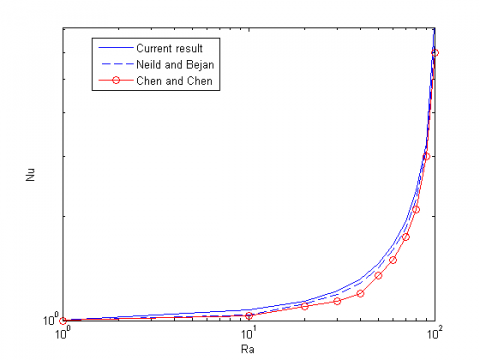Figure 2. Comparison between predicted results and those obtained by Neild & Bejan and Chen & Chen.

3. Results and Discussion

The stability of horizontal porous layer heated uniformly from below is analyzed using Forchheimer’s model, the system of governing equations and corresponding boundary conditions are non-linear partial differential equations depending on the Rayleigh number and Forchheimer’s number, the governing equations are written in dimensionless form using a suitable set of dimensionless variables, and then solved using a finite difference scheme. Two dimensionless groups are found to describe the problem; namely Rayleigh number which represent the buoyancy forces to viscose forces and the second the Forchhiemer’s number which represent the pore spaces.

3.1 Forchhiemer number effect

The effect of Forchheimer’s inertial coefficient can be studied by using different values for Forchheimer’s inertial coefficient used in the governing equations. Results for velocity and temperature profiles $\psi$ and $\theta$  for different values of Forchhiemer’s Coefficient will be presented in the figure 3 for the cases of  Fr equal to 0 and 0.09 for unsteady state case, and for all figures Ra (Rayleigh number)=10. Fig.3 shows the transient  dimensionless streamline and temperature profile for Fr equal to 0 and 0.09 at t equal to 0.02, when the Forchheimer’s number is increasing the dimensionless streamline value decreases; this is due to the fact that by increasing Forchheimer’s number the pore space increases which increase the viscous effect by the fluid.

## 3a1.png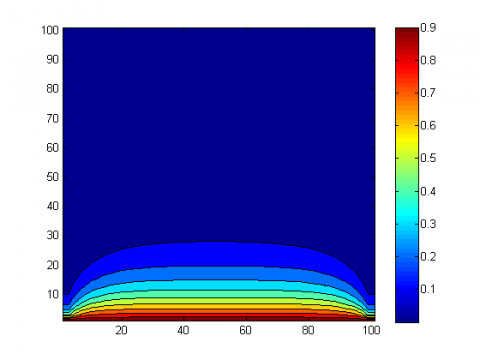## 3a2.png(a) Fr=0.0

## 3b1.png## 3b2.png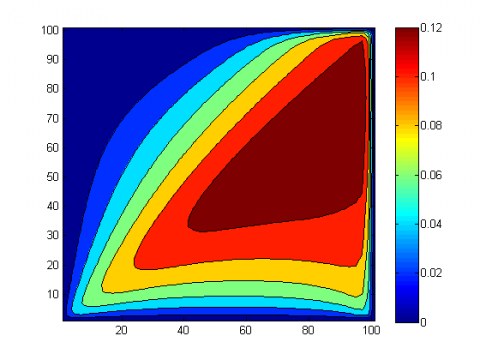(b) Fr=0.09

Figure 3. Typical dimensionless unsteady streamline and temperature patterns for Fr = 0.0 and 0.09 and Ra = 10.

The result also shows that Nu-mean decreases by increasing Fr due to the decreasing in the dimensionless streamline value in the porous media which decrease the convection heat transfer in the porous media that’s affect the value of Nusselt number.

Fig.4 shows the steady state dimensionless streamline and temperature profile for Fr equal to 0 and 0.09 at steady state condition, when the Forchheimer’s number is increasing the dimensionless streamline value decreases which decrease the convection heat transfer in porous media; this is due to the fact that by increasing Forchheimer’s number the pore space increases which increase the viscous effect by the fluid.

## 4a1.png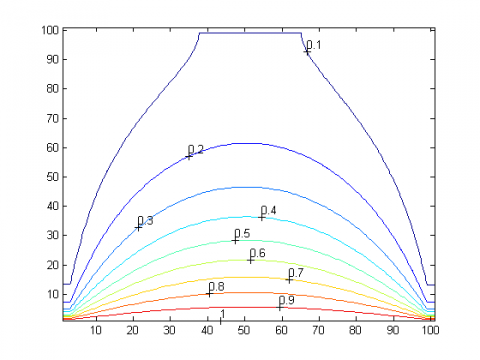## 4a2.png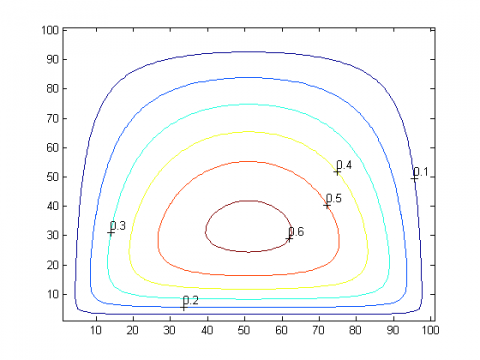(a) Fr=0.0

## 4b1.png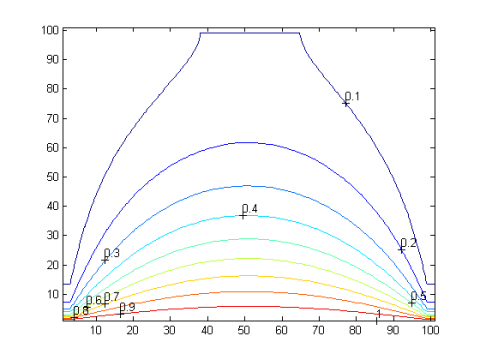## 4b2.png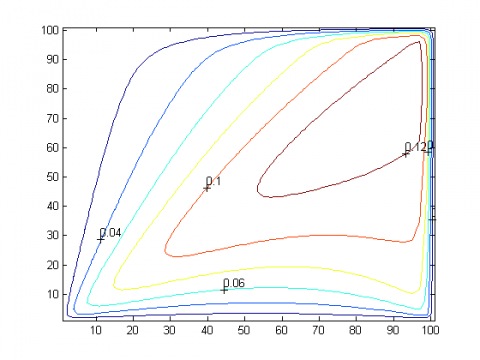(b) Fr=0.09

Figure 4. Typical dimensionless steady streamline and temperature patterns for Fr = 0.0 and 0.09 and Ra = 10.

3.2 Rayleigh number effect

The effect of Rayleigh number can be studied by using different values for Rayleigh number used in the governing equations. The Rayleigh number (Ra) for a fluid can be defined as dimensionless number associated with buoyancy driven flow (also known as free convection or natural convection) which describes the relationship between buoyancy and viscosity within a fluid.

## 5a1.png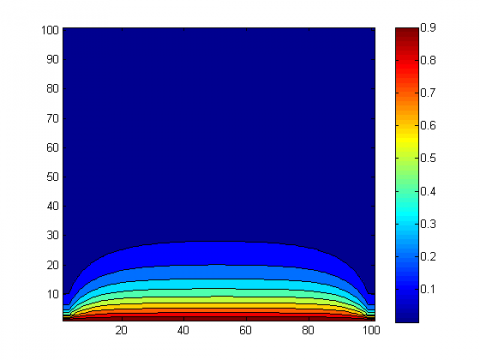## 5a2.png(a) Ra=1

## 5b1.png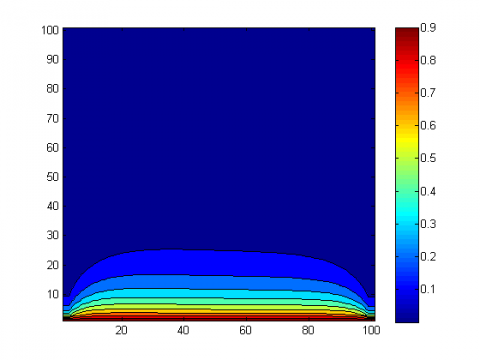## 5b2.png(b) Ra=50

Figure 5. Typical dimensionless unsteady streamline and temperature patterns for Ra = 10 and 50, and Fr = 0.001.

Fig. 5 shows the unsteady dimensionless temperature and streamline profile for Rayleigh number equal to 1 and 50 at t equal to 0.02 and Forchhiemer’s number equal to 0.001, changing Rayleigh number has a small effect on temperature profile at initial time, and there is a small different in dimensionless profile for different values of Rayleigh number; this is due to the effect of convection heat transfer which increase by increasing Rayleigh number. Changing Rayleigh number has an effect on dimensionless streamline value and profile at initial time; this is due to the effect of convection heat transfer which increases by increasing Rayleigh number over critical Rayleigh number.

## 6a1.png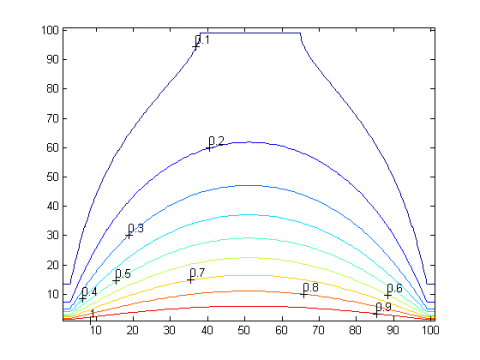## 6a2.png(a) Ra=1

## 6b1.png## 6b2.png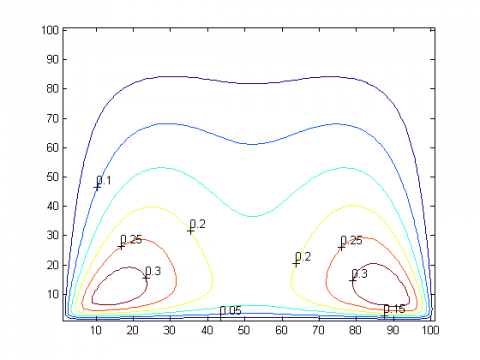(b) Ra=50

Figure 5. Typical dimensionless steady streamline and temperature patterns for Ra = 10 and 50, and Fr = 0.001.

Figure (5.13) shows the steady dimensionless temperature profile for Rayleigh number equal to 1 and 50 and Forchhiemer’s number equal to 0.001, changing Rayleigh number has an effect on temperature profile at steady state, and there is a noticeable difference in temperature profile for different values of Rayleigh number ; this is due to the effect of convection heat transfer which appears in the Rayleigh number equal to 50 which is over critical Rayleigh number it means that by increasing the Rayleigh number over critical Rayleigh number value the mode of heat transfer in porous media would be natural convection heat transfer. Also there is a noticeable difference in streamline profile for different values of Rayleigh number; this is due to the effect of convection heat transfer which appears in the Rayleigh number equal to 50 which is over critical Rayleigh number it means that by increasing the Rayleigh number over critical Rayleigh value the mode of heat transfer in porous media would be natural convection heat transfer and the streamline value will increase.

3.3 Critical Rayleigh number

Figures (6) shows the variation of critical Rayleigh number with Forchheimer’s number, the figure shows that as Forchhiemer’s number is increased the critical Rayleigh number also is increased due to the increase of permeability which decrease the velocity of the flow and so decrease the convection heat transfer effect, so increase the stability of fluid. From the obtained data a mathematical relationship between Rac and Fr is obtained:

Rac = -1504 $F r^{2}$ + 2055 Fr + 39.51      (12)

This equation describes the change of critical Rayleigh number with respect to Forchheimer’s number we notice that when Forchhiemer’s number equal to zero which represent a Darcy model the critical Rayleigh number equal to 39.51 which is almost the exact value as stated in references.

## 6.jpgFigure 6. Variation of critical Rayleigh number with Forchheimer’s number.

3.4 Nusselt number

## 7.jpg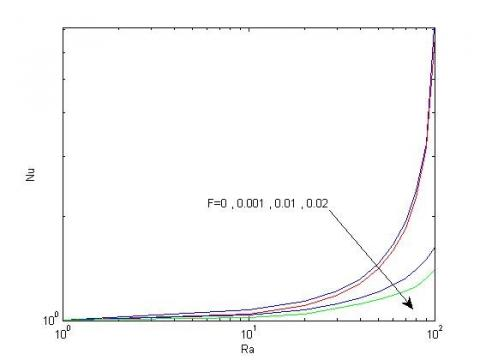Figure 7. Variation of Nusselt number with Rayleigh number for different Forchhiemer’s number.

Figure (7) shows the variation of Nusselt number with Rayleigh number for different values of Forchheimer’s Number Fr=0,0.02,0.05&0.09 the figure shows a proportional relationship between Rayleigh number and Nusselt number, when Rayleigh number increased Nusselt number also increased; this is due to the increase of the convection heat transfer value for small values of Rayleigh number Nusselt number equal one this result obtained when the heat transfer in porous media only conduction, but when Rayleigh number increased over critical value Nusselt number increased;Due to the convection heat transfer.

4. Conclusions

In this paper, the problem of stability of horizontal porous layer heated from below using Forchheimer’s model had been studied, parameters which directly affect the dimensionless velocity and temperature have been highlighted and discussed ending the following conclusions:

(1) The increase of the Rayleigh number over a critical value has found to change the heat transfer mode from conduction heat transfer to convection heat transfer.

(2) The increase of the Rayleigh number has found to increase the fluid velocity and also increase heat transfer in the porous layer.

(3) The Forchheimer’s number effect highly arise for high speeds of for high Reynolds number also its represent the drag on particles from inertia.

(4) The increase Forchheimer number has found to decrease the ability of fluid to move so the convection heat transfer in porous layer decreases.

(5) The Forchheimer’s inertial coefficient depends on the type of porous media also it has a large effect on velocity and a small effect on temperature.

Nonmenclature
 $u$ The velocity in x direction. [m/s] $v$ The velocity in y direction. [m/s] $T(x)$ A variable surface temperature [K] $T_{m}$ The fluid temperature [K] $g$ Gravitational acceleration. [m/s2] $K$ Permeability. [m2] $\mathrm{C}_{\mathrm{F}}$ Forchheimer constant. $P$ Pressure [Pa] $T$ Temperature. [K] $W$ The length in x-direction. [m] $H$ The length in y-direction. [m] $k_{a}$ Thermal conductivity of the porous media and fluid. [W/m.K] $\mathrm{c}_{\mathrm{p}}$ Specific heat at constant pressure. [kJ/kg.K] ​$F r$ Forchheimer number. ​Ra The Darcy-modified Rayleigh number. Greek symbols $\mu$ Absolute viscosity. [kg/m.s] $\rho$ The fluid density. [kg/ m3] $\sigma$ Electrical conductivity. $[\mathrm{m} \Omega / \mathrm{m}]$ $\beta$ Coefficient of thermal expansion. $\left[\mathrm{K}^{-1}\right]$ $\psi$ Streamline function. $\Psi$ Dimensionless stream function $\alpha_{\mathrm{a}}$ Thermal diffusivity. [m2/s] $v$ Dynamic viscosity. [m2/s]
References

1. Horton C. W. and Rogers Jr., F. T., Convection Currents in a Porous Medium, Journal of Applied Physics, 16(6), 367-370, 1945. DOI: 10.1063/1.1707601.

2. H. M. Duwairi, Radiation Effects on Mixed Convection over a Nonisothermal Cylinder and Sphere in a Porous Media, Journal of Porous Media, vol. 9, no. 3, pp. 251- 259, 2006. DOI: 10.1615/jpormedia.v9.i3.60.

3. H. M. Duwairi and Y. Al-Kablawi, Mhd-Conjugate Mixed Convection Heat Transfer over a Vertical Hollow Cylinder Embedded in a Porous Medium, Int. J. of Heat and Technology, vol. 24, no. 1, pp. 123-128, 2006.

4. H. M. Duwairi, T. K. Aldoss and M. H. Jarrah, Nonsimilarity Solutions for Non-Darcy Mixed Convection from Horizontal Surfaces in a Porous Medium, Heat and Mass Transfer, vol. 33, pp. 149-156, 1997. DOI: 10.1007/s002310050172.

5. Lapwood, E. R., Convection of a Fuid in a Porous Medium, Proc. Camb. Philos. Soc, 44, 508–521, 1948. DOI: 10.1017/s030500410002452x.

6. Katto, Y. and Masuoka, Y., Criterion for the Onset of Convective Flow in a Fluid in a Porous Medium, International Journal of Heat and Mass Transfer, 10(3), 297-309, 1967. DOI: 10.1016/0017-9310(67)90147-0.

7. Chen, F. and Chen, C. F., Natural Convection in Superposed Fluid and Porous Layers, Journal of Fluid Mechanics, 234, 97-119, 1992. DOI: 10.1017/ s0022112092000715.

8. Prasad, V., Flow Instabilities and Heat Transfer in Fluid Overlying Horizontal Porous Layers, Experimental Thermal and Fluid Science, 6 (2), 135–146, 1993. DOI: 10.1016/0894-1777(93)90023-C.

9. Steven S., Experimental Study of Free Convection in Fluid Porous Layers Heated from Below. Master’s thesis, University of Minnesota, 2006.

10. Nield, D., Bejan, A., Convection in Porous Media, Springer, 3rd ed, 2006. DOI: 10.1007/978-1-4614-5541- 7.